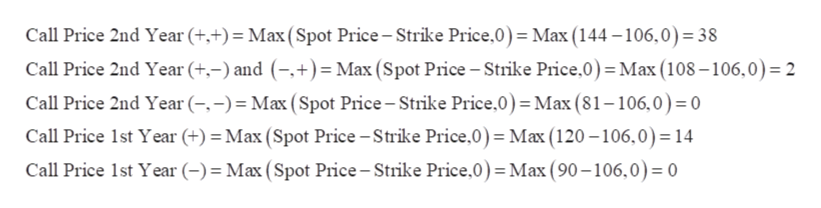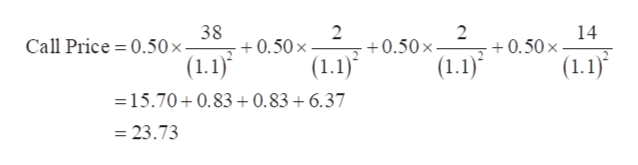# please answer c) only Question 1. Consider a two-step binomial tree, where a stock that pays no dividends has current price 100, and at each time step can increase by 20% or decrease by 10%. The possible values at times T = 2 are thus 144, 108 and 81. The annually compounded interest rate is 10%.a) Calculate the price of a two-year 106-strike European put using risk-neutral probabilities.b) Calculate the price of a two-year 106-strike European put using replication.c) Calculate the price of a two-year 106-strike American put using replication, and hence verify that the American put has price strictly greater than the European.d) Calculate the prices of a two-year 86-strike European put and American put. What is different from part (c)?

Question
8 views

Question 1. Consider a two-step binomial tree, where a stock that pays no dividends has current price 100, and at each time step can increase by 20% or decrease by 10%. The possible values at times T = 2 are thus 144, 108 and 81. The annually compounded interest rate is 10%.

a) Calculate the price of a two-year 106-strike European put using risk-neutral probabilities.

b) Calculate the price of a two-year 106-strike European put using replication.

c) Calculate the price of a two-year 106-strike American put using replication, and hence verify that the American put has price strictly greater than the European.

d) Calculate the prices of a two-year 86-strike European put and American put. What is different from part (c)?

check_circle

Step 1

c.

Strike Price = 106

Spot or Current Price = 100

Call Price = 23.73

Calculation of Price of a 2-year 106-strike American put is as follows:

Step 2

Working Note:

Strike Price = 106

Spot or Current Price = 100

Calculation of American Call Price in different scenarios is as follows:help_outlineImage TranscriptioncloseCall Price 2nd Year (+,+) = Max (Spot Price-Strike Price,0) = Max (144-106,0) = 38 Call Price 2nd Year (+,-) and (-,+) = Max (Spot Price-Strike Price,0) = Max (108-106,0)= 2 Call Price 2nd Year (-,-) Max (Spot Price-Strike Price,0) = Max (81-106,0) = 0 Call Price 1st Year (+) = Max (Spot Price -Strike Price,0) = Max (120 -106,0) = 14 Call Price 1st Year (-) Max (Spot Price-Strike Price,0) = Max (90-106,0) = 0 fullscreen
Step 3

Probability ...help_outlineImage Transcriptionclose38 0.50 x (1.1) 2 2 +0.50x 14 +0.50x Call Price 0.50x - (1.1) (1.1) (1.1) 15.70+0.83+ 0.83 +6.37 -23.73 fullscreen

### Want to see the full answer?

See Solution

#### Want to see this answer and more?

Solutions are written by subject experts who are available 24/7. Questions are typically answered within 1 hour.*

See Solution
*Response times may vary by subject and question.
Tagged in